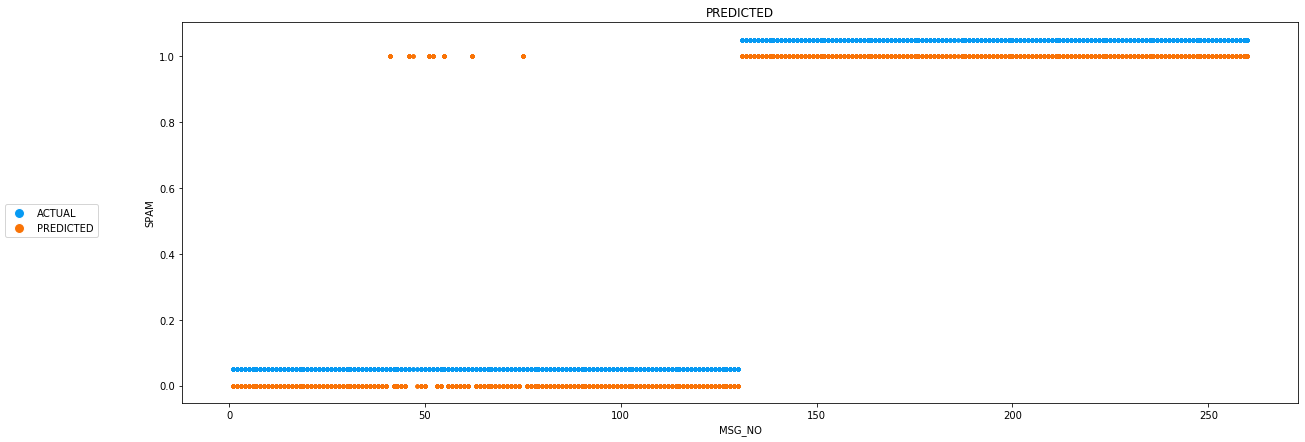# Naive Bayes Spam Filter¶

### 1.0.0 Import Packages¶

In :
import pandas as pd
from sklearn.metrics import accuracy_score
import seaborn as sns
import matplotlib.pyplot as plt
from matplotlib.lines import Line2D
import numpy as np
from sklearn.model_selection import train_test_split
from collections import defaultdict

In :
f_train_feat = "data/train-features.txt"
f_train_label = "data/train-labels.txt"
f_test_feat = "data/test-features.txt"
f_test_label = "data/test-labels.txt"
cols = ['MSG_NO','WORD_NO','CURR_FREQ']
output = ['SPAM']


### 2.1.0 Preprecess raw data¶

In :
#import dataset


In :
df_train_feat['SPAM'] = -1
df_test_feat['SPAM'] = -1
df_test_feat['PREDICTED SPAM'] = -1

In :
for i in range (0,df_train_label.shape):
df_train_feat.loc[df_train_feat.MSG_NO == i+1,'SPAM'] = df_train_label.iloc[i]['SPAM']

for i in range (0,df_test_label.shape):
df_test_feat.loc[df_test_feat.MSG_NO == i+1,'SPAM'] = df_test_label.iloc[i]['SPAM']


### 3.1.0 Naive Bayes approach¶

• probability for a set of samples : $P(\vec{samples}|class) = \prod_{i=1}^{n}P(sample_{i}|class)$
• conditional probability for a class given samples : $P(class_{i} | \vec{samples}) = \dfrac{P(class_{i})P(\vec{samples} | class_i)}{\displaystyle\sum_{i=1}^{n}{P(class_i)P(\vec{samples}|class_i)}}$
In :
#helper functions
def p_xi_spam(df,xi):
total = df[df['SPAM'] == 1]['MSG_NO'].nunique()
n_spam_xi = 0
for i in df[df['SPAM'] == 1]['MSG_NO'].unique():
if i in df[df['WORD_NO'] == xi]['MSG_NO'].values:
n_spam_xi+=1
return n_spam_xi/total

def p_xi_ham(df,xi):
total = df[df['SPAM'] == 0]['MSG_NO'].nunique()
n_ham_xi = 0
for i in df[df['SPAM'] == 0]['MSG_NO'].unique():
if i in df[df['WORD_NO'] == xi]['MSG_NO'].values:
n_ham_xi+=1
return n_ham_xi/total

def p_spam(df):
total = df['MSG_NO'].nunique()
n_spam = df[df['SPAM'] == 1]['MSG_NO'].nunique()
return n_spam/total

def p_ham(df):
total = df['MSG_NO'].nunique()
n_ham = df[df['SPAM'] == 0]['MSG_NO'].nunique()
return n_ham/total

def populate_dict(df):
sdict = defaultdict(int)
hdict = defaultdict(int)
for index,row in df.iterrows():
if row['SPAM'] == 1:
sdict[row['WORD_NO']] += row['CURR_FREQ']
else:
hdict[row['WORD_NO']] += row['CURR_FREQ']
return sdict,hdict

def predict_naivebayes(df_train_feat,df_test_feat,s_alpha=1.0,s_beta=2.0):
msgno = 0
p_spam = 0
p_ham = 0

sdict = defaultdict(int)
hdict = defaultdict(int)
sdict,hdict = populate_dict(df_train_feat)

spam_length = df_train_label[df_train_label['SPAM'] == 1].shape
ham_length = df_train_label[df_train_label['SPAM'] == 0].shape

test_spam_length = df_test_label[df_test_label['SPAM'] == 1].shape
test_ham_length = df_test_label[df_test_label['SPAM'] == 0].shape

test_p_spam = (test_spam_length /  test_spam_length + test_ham_length )
test_p_ham = (test_ham_length /  test_spam_length + test_ham_length )

# for each word of each message
for index,row in df_test_feat.iterrows():
if (msgno != row["MSG_NO"]):
p_spam = 0
p_ham = 0

# laplace smoothing
p_x_spam = ( sdict[row["WORD_NO"]] + s_alpha ) / (spam_length + s_beta )
p_x_ham = ( hdict[row["WORD_NO"]] + s_alpha ) / (ham_length + s_beta )

p_spam += ( np.log( p_x_spam ) * row["CURR_FREQ"] )
p_ham += ( np.log( p_x_ham ) * row["CURR_FREQ"] )

msgno = row["MSG_NO"]

if p_spam + np.log(test_p_spam) > p_ham + np.log(test_p_ham):
else:

return df_test_feat


### 4.1.0 Classifying messages¶

In :
# generate test msgs
df_test_feat = predict_naivebayes(df_train_feat,df_test_feat)
df_test_feat

Out:
MSG_NO WORD_NO CURR_FREQ SPAM PREDICTED SPAM
0 1 8 1 0 0
1 1 9 1 0 0
2 1 17 1 0 0
3 1 19 1 0 0
4 1 29 1 0 0
... ... ... ... ... ...
27974 260 1512 1 1 1
27975 260 1624 1 1 1
27976 260 1967 1 1 1
27977 260 1974 2 1 1
27978 260 1991 1 1 1

27979 rows × 5 columns

### 5.1.0 Visualizing Results¶

In :
fig, arr = plt.subplots(nrows=1, ncols=1, sharex=False, sharey=True)
fig.set_figwidth(20)
fig.set_figheight(7)
legend_elements = [Line2D(, , marker='o', color='w', label='ACTUAL',
markerfacecolor='xkcd:azure', markersize=10),
Line2D(, , marker='o', color='w', label='PREDICTED',
markerfacecolor='xkcd:orange', markersize=10)
]

fig.legend(handles=legend_elements, loc='center left')
arr.plot(df_test_feat['MSG_NO'],df_test_feat['PREDICTED SPAM'],'.',c='xkcd:orange')
arr.plot(df_test_feat['MSG_NO'],df_test_feat['SPAM']+0.05,'.',c='xkcd:azure')
arr.set_title('PREDICTED')
arr.set_xlabel('MSG_NO')
arr.set_ylabel('SPAM')
fig.show()### 6.1.0 Accuracy Score¶

In :
match = df_test_feat[(df_test_feat['SPAM'] == df_test_feat['PREDICTED SPAM'])]['MSG_NO'].nunique()
total = df_test_feat['MSG_NO'].nunique()
accuracy = match/total*100
df_accuracy = pd.DataFrame([[match,total,accuracy]],columns=['MATCHES','TOTAL MESSAGES','ACCURACY SCORE'])
df_accuracy

Out:
MATCHES TOTAL MESSAGES ACCURACY SCORE
0 252 260 96.923077